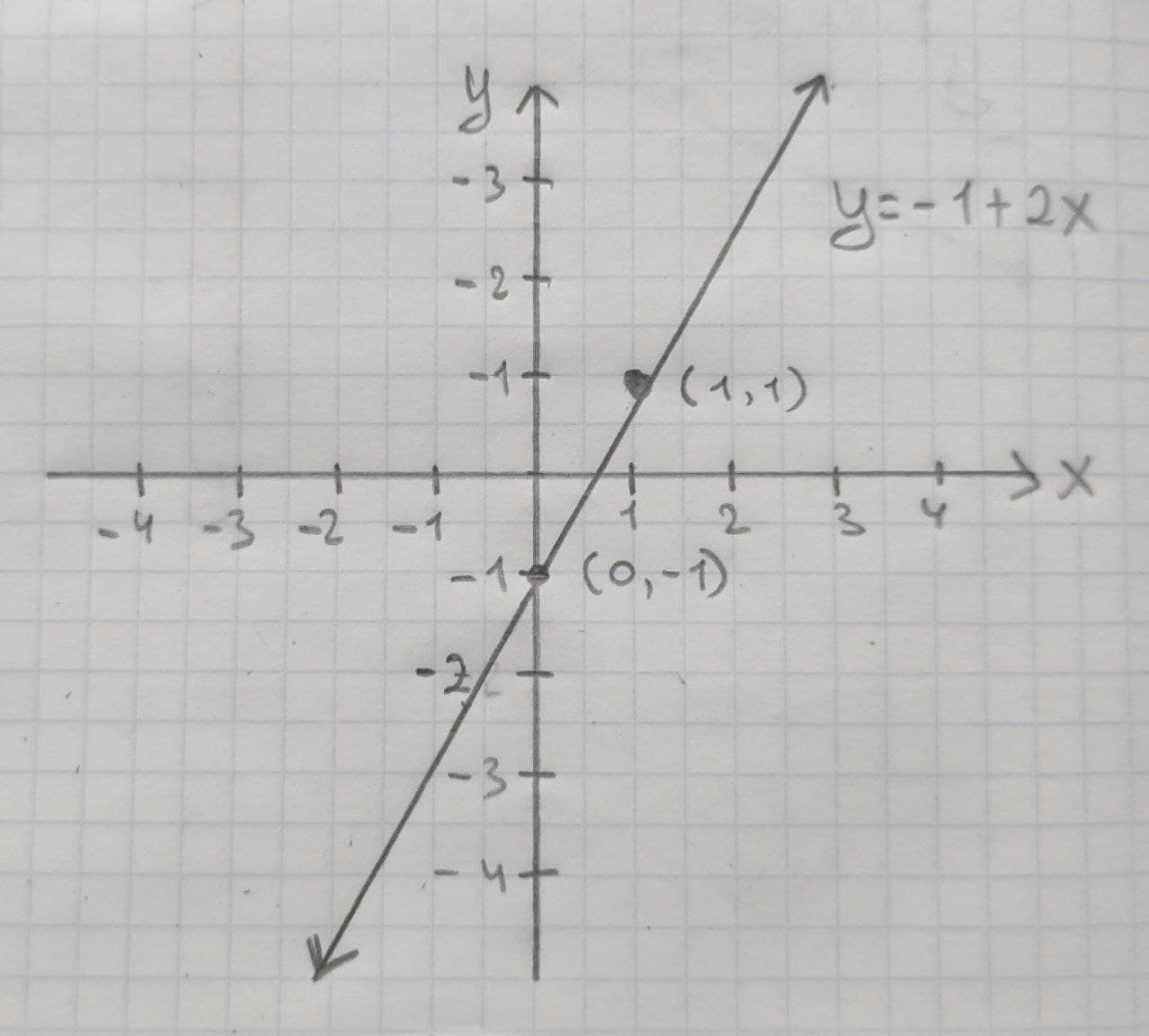# Given linear equation y = -1+2x a. find the y-intercept and slope. b. determine whether the line slopes upward, slopes downward, or is horizontal, without graphing the equation. c. use two points to graph the equation.CheemnCatelvew 2021-01-19 Answered
Given linear equation y = -1+2x
a. find the y-intercept and slope.
b. determine whether the line slopes upward, slopes downward, or is horizontal, without graphing the equation.
c. use two points to graph the equation.

• Questions are typically answered in as fast as 30 minutes

### Plainmath recommends

• Get a detailed answer even on the hardest topics.
• Ask an expert for a step-by-step guidance to learn to do it yourself.Mayme

(a) The linear equation is y=-1+2x.
In a linear equation $$\displaystyle{y}={b}_{{0}}+{b}_{{1}}{x}$$ the constant $$\displaystyle{b}_{{1}}$$ be the slope and $$\displaystyle{b}_{{0}}$$ be the y-intercept form and x is the independent variable and y is the independent variable.
Comparing the given equation with the general form of linear equation the slope of the equation is 4 and the y-intercept is 3. Thus, the slope of the linear equation is $$\displaystyle{b}_{{1}}={2}$$ and the y-intercept is $$\displaystyle{b}_{{0}}=-{1}$$.
(b) In a linear equation $$\displaystyle{y}={b}_{{0}}+{b}_{{1}}{x}$$ the constant $$\displaystyle{b}_{{1}}$$ be the slope and $$\displaystyle{b}_{{0}}$$ be the y-intercept form and x is the independent variable and y is the independent variable.
It is known that, the slope of the linear equation $$\displaystyle{y}={b}_{{0}}+{b}_{{1}}{x}$$ is upward if $$\displaystyle{b}_{{1}}{>}{0}$$, the slope of the linear equation $$\displaystyle{y}={b}_{{0}}+{b}_{{1}}{x}{y}={b}_{{0}}+{b}_{{1}}{x}$$ is downward if $$\displaystyle{b}_{{1}}{<}{0}$$ and the slope of the linear equation $$\displaystyle{y}={b}_{{0}}+{b}_{{1}}{x}$$ is horizontal if $$\displaystyle{b}_{{1}}={0}.$$
Thus, in the given equation $$\displaystyle{y}=-{1}+{2}{x},{b}_{{1}}={2}{<}{0}.$$
Thus, the slope is upward.
(c) The linear equation is y=-1+2x.
Graph the line:
The two points $$\displaystyle{\left({x}_{{1}}, {y}_{{1}}\right)}{\quad\text{and}\quad}{\left({x}_{{2}}, {y}_{{2}}\right)}$$ on the given line are obtained:
If x = 0,
$$\displaystyle{y}=-{1}+{\left({2}\times{0}\right)}$$
y=-1
Thus, one point on the line is $$\displaystyle{\left({x}_{{1}}, {y}_{{1}}\right)}={\left({0},-{1}\right)}.$$
If x = 1
$$\displaystyle{y}=-{1}+{\left({2}\times{1}\right)}$$
y=1
Thus, the second point on the line is $$(x_2, y_2)=(1,1)$$.